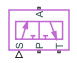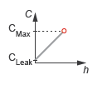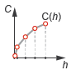# 3-Way Directional Valve (G)

Controlled valve with three ports in a gas network

•Libraries:
Simscape / Fluids / Gas / Valves & Orifices / Directional Control Valves

## Description

The 3-Way Directional Valve (G) block represents a valve with three gas ports (P, A, and T) and two flow paths between PA and AT. The paths each run through an orifice of variable width. The input signal specified at port S controls the position of the spool. The valve closes when the spool covers the orifice opening.

In a representative system, the P port connects to the pump, the T port connects to the tank, and the A port connects to a single-acting actuator. Opening the PA flow path, while closing AT, allows the pump to pressurize the actuator. Opening the AT flow path, while closing PA, allows the tank to absorb the excess pressure from the actuator, freeing the shaft to translate in reverse.

This image shows a common use for a three-way directional valve in a physical system:The flow can be laminar or turbulent, and it can reach up to sonic speeds. The maximum velocity happens at the throat of the valve where the flow is narrowest and fastest. The flow chokes and the velocity saturates when a drop in downstream pressure can no longer increase the velocity. Choking occurs when the back-pressure ratio reaches the critical value characteristic of the valve. The block does not capture supersonic flow.

### Valve Positions

The valve is continuously variable and it shifts smoothly between one normal and two working positions.

When the instantaneous displacement of the spool at port S is zero, the valve reverts to the normal position where it is no longer operating. Unless the lands of the spool are at an offset to their orifices, the valve is fully closed.

The working positions are the positions the valve moves to when the spool is maximally displaced, in either positive or negative direction, from the normal position. One orifice will then generally be closed and the other open to full capacity. Image I shows a valve where the displacement is in the negative direction, the PA orifice is closed, and the AT orifice is open. Image II shows a valve where the displacement is in the positive direction, the PA orifice is open, and the AT orifice is closed.The spool displacement that puts the valve in its working position depends on the offsets of the lands on the spool. The parameters in the Valve Opening Fraction Offsets section specify the block constants for the spool displacements of the ports.

Orifice Opening Fractions

Between valve positions, the opening of an orifice depends on where the land of the spool is, relative to the rim. This distance is the orifice opening, and the block normalizes this distance so that its value is a fraction of the maximum distance at which the orifice is fully open. The normalized variable is the orifice opening fraction.

The orifice opening fractions range from `-1` when the valve is in the position shown in image I to `+1` when the valve is in the position shown in image II.

The block calculates the opening fractions from the spool displacement and opening fraction offset. The displacement and offset are unitless fractions of the maximum land-orifice distance.

The opening fraction of the PA orifice is:

`${h}_{PA}={H}_{PA}+x.$`

The opening fraction of the AT orifice is:

`${h}_{AT}={H}_{AT}-x.$`

In both equations:

• h is the opening fraction of the orifice specified by the subscript PA or AT. If the calculation returns a value outside of the range ```0 - 1```, the block uses the nearest limit.

• H is the opening fraction offset for the orifice. The parameters in the Valve Opening Fraction Offsets section specify the opening fraction offsets. To allow for unusual valve configurations, the block imposes no limit on their values, although generally these will fall between `-1` and `+1`.

• x is the normalized instantaneous displacement of the spool, specified by a physical signal at port S. To compensate for extreme opening fraction offsets, there is no limit on the signal value. The value is typically between `-1` and `+1`.

Opening Fraction Offsets

By default, the valve is fully closed when its control displacement is zero. In this state, the valve is zero-lapped.

You can offset the lands of the spool to model an underlapped or overlapped valve. Underlapped valves are partially open in the normal position. Overlapped valves are fully closed slightly beyond the normal position. The figure shows how the orifice opening fractions vary with the instantaneous spool displacement:

• Image I: A zero-lapped valve. The opening fraction offsets are both zero. When the valve is in the normal position, the lands of the spool completely cover both orifices.

• Image II: An underlapped valve. The opening fraction offsets are both positive. When the valve is in the normal position, the lands of the spool partially cover both orifices.

• Image III: An overlapped valve. The opening fraction offsets are both negative. The lands of the spool completely cover both orifices not only in the normal position but over a small region of spool displacements around it.### Valve Parameterizations

The block behavior depends on the Valve parametrization parameter:

• `Cv flow coefficient` — The flow coefficient Cv determines the block parameterization. The flow coefficient measures the ease with which a gas can flow when driven by a certain pressure differential. 

• `Kv flow coefficient` — The flow coefficient Kv, where ${K}_{v}=0.865{C}_{v}$, determines the block parameterization. The flow coefficient measures the ease with which a gas can flow when driven by a certain pressure differential. 

• `Sonic conductance` — The sonic conductance of the resistive element at steady state determines the block parameterization. The sonic conductance measures the ease with which a gas can flow when choked, which is a condition in which the flow velocity is at the local speed of sound. Choking occurs when the ratio between downstream and upstream pressures reaches a critical value known as the critical pressure ratio. 

• `Orifice area` — The size of the flow restriction determines the block parametrization. 

### Opening Characteristics

The flow characteristic relates the opening of the valve to the input that produces it, which is often the spool travel. The block expresses the opening as a sonic conductance, flow coefficient, or restriction area, depending on the setting of the Valve parameterization parameter. The control input is the orifice opening fraction, a function of the spool displacement specified at port S.

The flow characteristic is normally given at steady state, with the inlet at a constant, carefully controlled pressure. The flow characteristic depends only on the valve and can be linear or nonlinear. To capture the flow characteristics, use the Opening characteristic parameter:

• `Linear` — The measure of flow capacity is a linear function of the orifice opening fraction. As the opening fraction rises from `0` to `1`, the measure of flow capacity scales from the specified minimum to the specified maximum.• `Tabulated` — The measure of flow capacity is a general function, which can be linear or nonlinear, of the orifice opening fraction. The function is specified in tabulated form, with the independent variable specified by the Opening fraction vector### Numerical Smoothing

When the parameter is `Linear`, and the Smoothing factor parameter is nonzero, the block applies numerical smoothing to the orifice opening fraction. Enabling smoothing helps maintain numerical robustness in your simulation.

### Leakage Flow

The leakage flow ensures that no section of a fluid network becomes isolated. Isolated fluid sections can reduce the numerical robustness of the model, slow the rate of simulation and, in some cases, cause the simulation to fail. The Leakage flow fraction parameter represents the leakage flow area in the block as a small number greater than zero.

### Composite Structure

This block is a composite component comprising two instances of the Orifice (G) block, one connected between ports P and A and a second block between A and T. Refer to the Orifice (G) block for more detail on the valve parameterizations and block calculations.

### Assumptions and Limitations

• The `Sonic conductance` setting of the Valve parameterization parameter is for pneumatic applications. If you use this setting for gases other than air, you may need to scale the sonic conductance by the square root of the specific gravity.

• The equation for the `Orifice area` parameterization is less accurate for gases that are far from ideal.

• This block does not model supersonic flow.

## Ports

### Input

expand all

Instantaneous displacement of the control member against its normal unactuated position, specified as a physical signal. The block normalizes the displacement against the maximum position of the control member that required to open the orifice fully. The signal is unitless and its instantaneous value is typically in the range between `-1` and `+1`.

### Conserving

expand all

Gas conserving port associated with the opening through which the flow enters or exits the valve.

Gas conserving port associated with the opening through which the flow enters or exits the valve.

Gas conserving port associated with the opening through which the flow enters or exits the valve.

## Parameters

expand all

Method to calculate the mass flow rate.

• `Cv flow coefficient` — The flow coefficient Cv determines the block parameterization.

• `Kv flow coefficient` — The flow coefficient Kv, where ${K}_{v}=0.865{C}_{v}$, determines the block parameterization.

• `Sonic conductance` — The sonic conductance of the resistive element at steady state determines the block parameterization.

• `Orifice area` — The size of the flow restriction determines the block parametrization.

Method the block uses to calculate the opening area of the valve. The linear setting treats the opening area as a linear function of the orifice opening fraction. The tabulated setting allows for a general, nonlinear relationship you can specify in tabulated form.

Vector of control signal values at which to specify the chosen measure of flow capacity: Sonic conductance vector, Cv coefficient vector, Kv coefficient vector, or Orifice area vector. The control signal is between `0` and `1`, with each value corresponding to an opening fraction of the resistive element. The greater the value, the greater the opening and easier the flow.

The opening fractions must increase monotonically across the vector from left to right. The size of this vector must be the same as the Sonic conductance vector, Cv coefficient vector, Kv coefficient vector, or Orifice area vector parameter.

#### Dependencies

To enable this parameter, set Opening characteristic to `Tabulated`.

Value of the Cv flow coefficient when the restriction area available for flow is at a maximum. This parameter measures the ease with which the gas traverses the resistive element when driven by a pressure differential.

#### Dependencies

To enable this parameter, set Valve parameterization to `Cv flow coefficient`, and Opening characteristic to `Linear`.

Ratio between the inlet pressure, pin, and the outlet pressure, pout, defined as $\left({p}_{in}-{p}_{out}\right)/{p}_{in}$ where choking first occurs. If you do not have this value, look it up in table 2 in ISA-75.01.01 . Otherwise, the default value of 0.7 is reasonable for many valves.

#### Dependencies

To enable this parameter, set Valve parameterization to `Cv flow coefficient` or ```Kv flow coefficient```.

Ratio of the flow rate of the orifice when it is closed to when it is open.

#### Dependencies

To enable this parameter, set Opening characteristic to `Linear`.

Continuous smoothing factor that introduces a layer of gradual change to the flow response when the orifice is in near-open or near-closed positions. Set this parameter to a nonzero value less than one to increase the stability of your simulation in these regimes.

#### Dependencies

To enable this parameter, set Opening characteristic to `Linear`.

Vector of Cv flow coefficients. Each coefficient corresponds to a value in the Opening fraction vector parameter. This parameter measures the ease with which the gas traverses the resistive element when driven by a pressure differential. The flow coefficients must increase monotonically from left to right, with greater opening fractions representing greater flow coefficients. The size of the vector must be the same as the Opening fraction vector.

#### Dependencies

To enable this parameter, set Valve parameterization to `Cv flow coefficient`, and Opening characteristic to `Tabulated`.

Value of the Kv flow coefficient when the restriction area available for flow is at a maximum. This parameter measures the ease with which the gas traverses the resistive element when driven by a pressure differential.

#### Dependencies

To enable this parameter, set Valve parameterization to `Kv flow coefficient`, and Opening characteristic to `Linear`.

Vector of Kv flow coefficients. Each coefficient corresponds to a value in the Opening fraction vector parameter. This parameter measures the ease with which the gas traverses the resistive element when driven by a pressure differential. The flow coefficients must increase monotonically from left to right, with greater opening fractions representing greater flow coefficients. The size of the vector must be the same as the Opening fraction vector parameter.

#### Dependencies

To enable this parameter, set Valve parameterization to `Kv flow coefficient`, and Opening characteristic to `Tabulated`.

Value of the sonic conductance when the cross-sectional area available for flow is at a maximum.

#### Dependencies

To enable this parameter, set Valve parameterization to `Sonic conductance`, and Opening characteristic to `Linear`.

Pressure ratio at which flow first begins to choke and the flow velocity reaches its maximum, given by the local speed of sound. The pressure ratio is the outlet pressure divided by inlet pressure.

#### Dependencies

To enable this parameter, set Valve parameterization to `Sonic conductance`, and Opening characteristic to `Linear`.

Empirical value used to more accurately calculate the mass flow rate in the subsonic flow regime.

#### Dependencies

To enable this parameter, set Valve parameterization to `Sonic conductance`.

Temperature at standard reference atmosphere, defined as 293.15 K in ISO 8778.

#### Dependencies

To enable this parameter, set Valve parameterization to `Sonic conductance`.

Density at standard reference atmosphere, defined as 1.185 kg/m3 in ISO 8778.

#### Dependencies

To enable this parameter, set Valve parameterization to `Sonic conductance`.

Vector of sonic conductances inside the resistive element. Each conductance corresponds to a value in the Opening fraction vector parameter. The sonic conductances must increase monotonically from left to right, with greater opening fractions representing greater sonic conductances. The size of the vector must be the same as the Opening fraction vector parameter.

#### Dependencies

To enable this parameter, set Valve parameterization to `Sonic conductance`, and Opening characteristic to `Tabulated`.

Vector of critical pressure ratios at which the flow first chokes, with each critical pressure ratio corresponding to a value in the Opening fraction vector parameter. The critical pressure ratio is the fraction of downstream-to-upstream pressures at which the flow velocity reaches the local speed of sound. The size of the vector must be the same as the Opening fraction vector parameter.

#### Dependencies

To enable this parameter, set Valve parameterization to `Sonic conductance`, and Opening characteristic to `Tabulated`.

Cross-sectional area of the orifice opening when the cross-sectional area available for flow is at a maximum.

#### Dependencies

To enable this parameter, set Valve parameterization to `Orifice area`, and Opening characteristic to `Linear`.

Correction factor that accounts for discharge losses in theoretical flows.

#### Dependencies

To enable this parameter, set Valve parameterization to `Orifice area`.

Vector of cross-sectional areas of the orifice opening. The values in this vector correspond one-to-one with the elements in the Opening fraction vector parameter. The first element of this vector is the orifice leakage area and the last element is the maximum orifice area.

#### Dependencies

To enable this parameter, set Valve parameterization to `Orifice area`, and Opening characteristic to `Tabulated`.

Pressure ratio at which flow transitions between laminar and turbulent flow regimes. The pressure ratio is the outlet pressure divided by inlet pressure. Typical values range from `0.995` to `0.999`.

Area normal to the flow path at the valve ports. The ports are assumed to be of the same size. The flow area specified here should ideally match those of the inlets of adjoining components.

Valve Opening Fraction Offsets

Opening fraction of the PA orifice when the value of the input signal is zero. The valve is then in the normal position. The opening fraction measures the distance of a land of the spool to its appointed orifice, here PA, normalized by the maximum such distance. It is unitless and generally between `0` and `1`.

Opening fraction of the AT orifice when the value of the input signal is zero. The valve is then in the normal position. The opening fraction measures the distance of a land of the spool to its appointed orifice, here AT, normalized by the maximum such distance. It is unitless and generally between `0` and `1`.

 ISO 6358-3. "Pneumatic fluid power – Determination of flow-rate characteristics of components using compressible fluids – Part 3: Method for calculating steady-state flow rate characteristics of systems". 2014.

 IEC 60534-2-3. "Industrial-process control valves – Part 2-3: Flow capacity – Test procedures". 2015.

 ANSI/ISA-75.01.01. "Industrial-Process Control Valves – Part 2-1: Flow capacity – Sizing equations for fluid flow underinstalled conditions". 2012.

 P. Beater. Pneumatic Drives. Springer-Verlag Berlin Heidelberg. 2007.